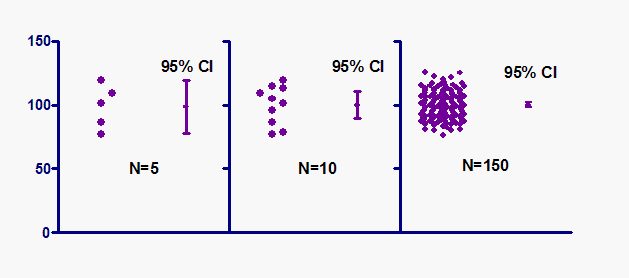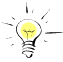## Please enable JavaScript to view this site.

 Navigation: PRINCIPLES OF STATISTICS > Confidence intervals Interpreting a confidence interval of a mean

## A confidence interval does not quantify variability

A 95% confidence interval is a range of values that you can be 95% certain contains the true mean of the population. This is not the same as a range that contains 95% of the values. The graph below emphasizes this distinction.

###The graph shows three samples (of different size) all sampled from the same population.

With the small sample on the left, the 95% confidence interval is similar to the range of the data. But only a tiny fraction of the values in the large sample on the right lie within the confidence interval. This makes sense. The 95% confidence interval defines a range of values that you can be 95% certain contains the population mean. With large samples, you know that mean with much more precision than you do with a small sample, so the confidence interval is quite narrow when computed from a large sample.Don't view a confidence interval and misinterpret it as the range that contains 95% of the values.

## A 95% chance of what?

It is correct to say that there is a 95% chance that the confidence interval you calculated contains the true population mean. It is not quite correct to say that there is a 95% chance that the population mean lies within the interval.

What's the difference?

The population mean has one value. You don't know what it is (unless you are doing simulations) but it has one value. If you repeated the experiment, that value wouldn't change (and you still wouldn't know what it is). Therefore it isn't strictly correct to ask about the probability that the population mean lies within a certain range.

In contrast, the confidence interval you compute depends on the data you happened to collect. If you repeated the experiment, your confidence interval would almost certainly be different. So it is OK to ask about the probability that the interval contains the population mean.

It is not quite correct to ask about the probability that the population mean is within the interval. It either is in the interval or it isn't. There is no chance about it. What you can say is that if you perform this kind of experiment many times, the confidence intervals would not all be the same, you would expect 95% of them to contain the population mean, you would expect 5% of the confidence intervals to not include the population mean, and you would never know whether the interval from a particular experiment contained the population mean or not.